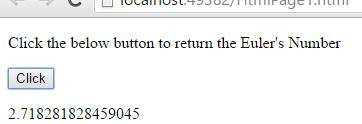# Euler's Number (E) in JavaScript

#### E Property (E - Euler's Number)

The number `e` is the one of the most imporatant numbers in mathematics, it was discovered by "Leonhard Euler" and it is often called "Euler's Number" after "Leonhard Euler". It is a famous irrational number`e` is found in many interesting areas, so it is worth learning  about. There are many ways to calculate the value of `e`, but none of them give an exact answer, because `e` is irrational number

In JavaScript, by using `e` property we can return the Euler's Number.

```<p>Click the below button to return the Euler's Number</p>
<input type="button" onclick="myNumber()" value="Click">
<p id="myId"></p>

<script>
function myNumber() {
document.getElementById("myId").innerHTML = Math.E;
}
</script>
```

In the above code snippet we have given `Id` as "`myId`"to the second `<p>` element in the HTML code. There is a function myNumber() in the`<script>`block which is connected to the onclick of the HTML button. We need to return the Euler's number for that we are using `E` property. Onclick of the button "Click" in the HTML code fires the function myNuber() in the `<script>` block at the same time `Math.E` property returns the Euler's number as output.

OUTPUTViews: 5432 | Post Order: 91